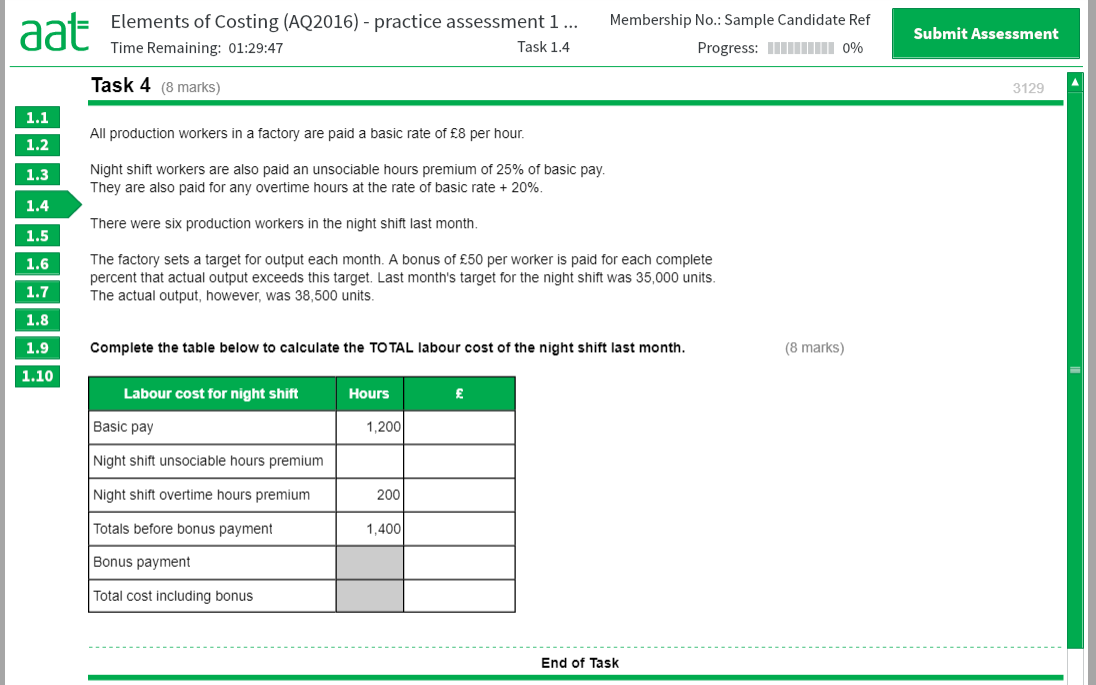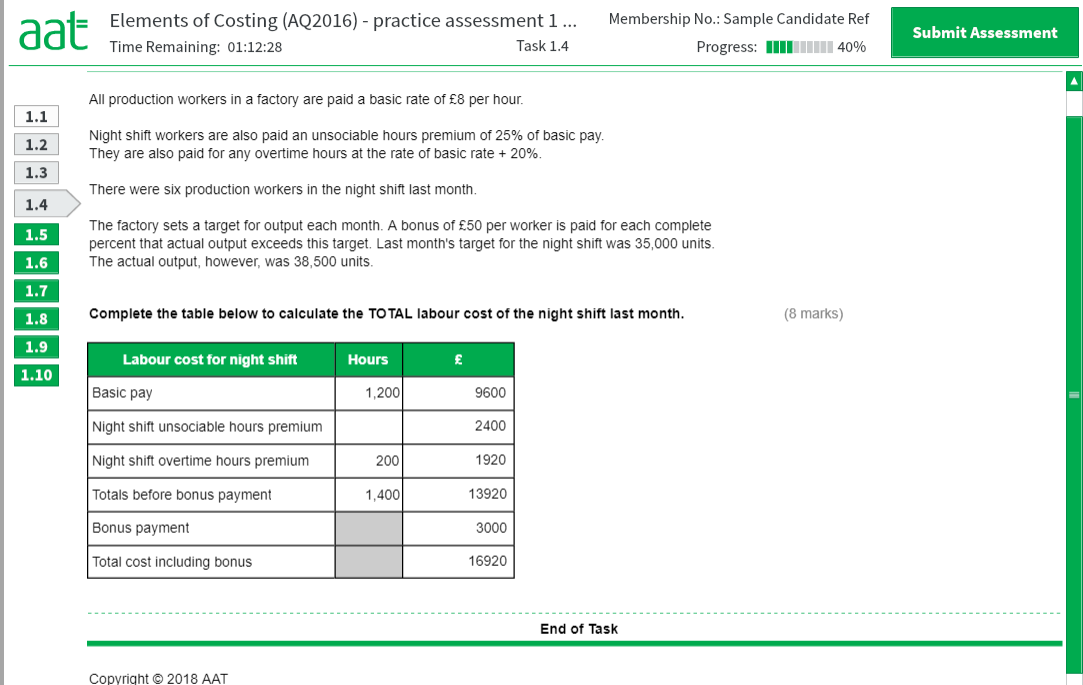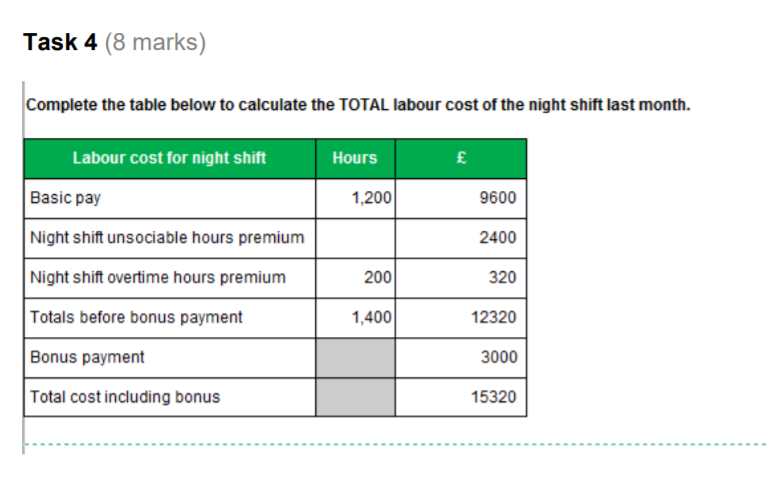# Is CBT practice assessment answer wrong for task in ELCO or I am missing something?

Hello,

I am preparing myself for ELCO assessment and there is a task I am not sure whether answer from AAT website is correct because it doesn't make sense to me. I would be very grateful if someone could review it and let me know where I am wrong.

Please see details below and main question after all pictures:So main difference is with how to calculate Night shift overtime hours premium.
My calculation: basic rate plus 20% which is 1.2 x 8 = £9.60 and this times 200 (9.60 x 200 = 1920)
AAT calculation include only premium so 0.2 x 8 = £1.60 and this times 200 (1.60 x 200 = 320).

What confuse me is the total hours is 1400 so that means overtime hours are not included in basic pay so seems like AAT wouldn’t pay basic rate for overtime hours at all (only premium). Is there something I am missing or practice CBT answers might be wrong and if this is the case what way I should calculate it on the exam if I receive similar question?

Thank you in advance for help

AAT Level 2. 2018 Distinction
AAT Level 3. 2018 Distinction
AAT Level 4. 2019 FSLC 100% MABU 93% MADC 82% PDSY 80%
• Thank you for your reply. I have read it and I now understand that if Task is asking to provide what will be overtime premium it needs to be 320 however I do not understand why in whole calculation the basic pay for overtime is not included. Why total of hours shows 1400 hours and basic pay 1200 -> that means 200 hours of overtime are not included in basic pay.. Maybe total should be 1200 and then 200 h overtime would be included in basic pay? This is what I am struggling with.
• I think you need to read again what I have sent you and in particular the concept behind the overtime
In simple words overtime is simply devided into basic pay(£8) and premium pay (£1.6)and this have been calculated accordingly
What you are looking for simply doesn't exist in this task as a separate calculation, because it has already been taken into consideration
AAT Level 2. 2018 Distinction
AAT Level 3. 2018 Distinction
AAT Level 4. 2019 FSLC 100% MABU 93% MADC 82% PDSY 80%
• Thank you dumutroba. That makes sense now.. whenever task will ask for premium this is all I will bother about and will not over complicate my thinking next time with looking for calculation I am not asked about. Thanks a lot.